# Quaternary (Base Four), the Neglected Radix (Number Base).

Quaternary /kwəˈtɜːrnəri/ is the easiest and simplest form of binary (base two) besides binary itself. By that I mean that quaternary is, like octal (base eight) and hexadecimal (base sixteen) and every other radix that is a power of two, an abbreviated notation for binary.

If that isn’t clear to you, please use this Wikipedia article to get up to speed on quaternary.

Quaternary is also the easiest and simplest form of any number base that is not that number base itself.

Quaternary is the smallest base that is moderately practical (just my opinion). Binary requires too many digits to write a number (again, just my opinion) to be practical. Ternary (base three) might be moderately practical except that three is an odd number, and therefore halving and doubling is messy, and iterated halving or doubling is even worse.

Quaternary, as pointed out by the Wikepedia article linked to above, is practical as a base because “four is the largest number within the subitizing range”. This just means that four dots can be always be instantly and confidently recognized as four dots whereas that cannot be said for a higher number of dots, such as five dots.

I think a great use for quaternary is as an easy alternative number base (an alternative to our everyday decimal counting system), in research, and for teaching students in school. Notice that I didn’t say ‘math research’, or ‘teaching math to students in school’. That was deliberate on my part. I think the study of quaternary, although great for researching and teaching math, may also be useful in other areas such as linguistics.

Heximal (base six) is sometimes taught in school, as is octal, and of course binary. Duodecimal (base twelve), also known as dozenal, and hexadecimal are less often seen in schools, maybe because teachers draw the line at bases so large that they need new symbols to represent them. Quaternary is never taught, as far as I know, and this seems to be a great pity, because it has the advantage of being the simplest number base.

Searching on the Internet yielded only https://www.mathsisfun.com/numbers/bases.html which does teach base four but only because it teaches every base from base two to base twelve. Base four sits there between base three and base five, and not far from base seven and even base eleven. That tells me a lot.

It is has only four numerals: 0, 1, 2, and 3. The addition table and the multiplication table is so small and easy that students can easily memorize them and then proceed to do arithmetic in quaternary.

## How to pronounce quaternary.

There are several ways that work well for pronouncing quaternary.

There are so few symbols that it is easy to replace them with new symbols and spoken names, and again, students can easily memorize them and count and do arithmetic in a truly ‘foreign’ quaternary. Not only would this prevent confusion with decimal, it makes the whole thing into an interesting and fun exercise in linguistics. It’s not clear whether a student’s ability to recall the decimal times table is affected by learning the times table of another base, but it does seem to be a common fear, and one way that fear can be allayed is by using an entirely separate set names and symbols for the numbers. And doing this is easy when you have only four numerals to rename.

The powers of four do not need renaming if my system of names of for all positive powers of two is raided for those powers of two that are also powers of four. This article includes an easy introduction to the naming system for powers of two: https://bartshmatthew.medium.com/how-a-math-teacher-should-pronounce-a-binary-number-1c41773df52f.

Here’s the easiest way to count in quaternary if you’ve never done it before, without any renaming. It’s very useful for thinking about base four without mastering any new names or symbols.

quaternary: 1, 2, 3, 10, 11, 12, 13, 20, 21…

(decimal translation: 1, 2, 3, 4, 5, 6, 7, 8, 9…)

Pronounced ‘one, two, three, one four, one four and one, one four and two, one four and three, two fours, two fours and one…’

Optionally getting a bit more sophisticated and dropping the ‘and’s and the plurals we have: ‘one, two, three, one four, one four one, one four two, one four three, two four, two four one…’

This is analogous to switching from decimal ‘two hundreds and one’ to ‘two hundred one’.

Here are some bigger quaternary numbers.

quaternary: 33, 100, 101, 102, 103, 110, 111, 112…

(decimal translation: 15, 16, 17, 18, 19, 20, 21, 22…)

Pronounced ‘three fours and three, one sixteen, one sixteen, one sixteen and one, one sixteen and two, one sixteen and three, one sixteen one four and one, one sixteen one four and two…’

The traces of decimal are obvious, in addition to the use of the symbols and names of the numerals, the names of the powers of four recall decimal: ‘sixteen’ means ‘six plus ten’, for example. It’s not fatal, but it is interesting to consider trying to get entirely away from decimal.

The most offensive trace of decimal is surely ‘sixteen’, and of course ‘sixty-four’ is even worse, and ‘two hundred and fifty-six’ worse still. A simple way forward is to instead say, ‘four squared’ or ‘two to the four’ instead of sixteen. Likewise instead of ‘sixty-four’ you could have ‘four cubed’ or ‘two to the six’, and instead of ‘two hundred fifty-six’ you could have ‘ four to the four’ or ‘two to the eight’ and so on. But if you try counting like that, you’ll soon see how confusing that is, especially when you can’t say ‘squared’ or ‘cubed’ because the number is too big for that.

That’s why I came up with my algorithm for names of powers of two described in https://bartshmatthew.medium.com/how-a-math-teacher-should-pronounce-a-binary-number-1c41773df52f.

## A more sophisticated system of pronunciation for quaternary.

Two is ‘ti’, four is ‘ni’, eight is ‘mi’, sixteen is ‘ri’, and so one ad infinitum according to the algorithm. Taking the powers of four only, gives us four is ‘ni’, sixteen is ‘ri’… ad infinitum.

Using that system, one pronounces

quaterary: 33, 100, 101, 102, 103, 110, 111, 112…

(decimal translation: 15, 16, 17, 18, 19, 20, 21, 22…)

as ‘three ni three, one ri, one ri one, one ri two, one ri three, one ri one ni, one ri one ni one one, one ri one ni two…’

That’s much better. Stopping at this point is an option, but if one wishes to remove the remaining stuff in common with decimal (and as I explained above, it may be important to be able to do so) one has only four numerals to rename. Here’s one way inspired by and designed to dovetail with the hexadecimal naming system that is so popular and entrenched ( by the way, my proposed optional new symbol set for hexadecimal are, starting at zero, J, Q, H, S, K, R, M, N, W, V, A, B, C, D, E, F. Thus fifteen is F, sixteen is QJ, seventeen is QQ and so on. Because A to F are retained with their present meanings, there is no conflict with the entrenched symbol set. But I digress)

0 becomes ‘J’ (‘jay’) (or optionally is unchanged, remaining 0 (‘zero))

1 becomes ‘Q’ (‘cue’)

2 becomes ‘H’ (‘aitch’)

3 becomes ‘S’ (‘ess’)

quaterary: 33, 100, 101, 102, 103, 110, 111, 112…

becomes quaternary: ‘SS, QJJ, QJQ, QJH, QJS, QQJ, QQQ, QQH…’

(decimal translation: 15, 16, 17, 18, 19, 20, 21, 22…)

pronounced as ‘three ni three, one ri, one ri one, one ri two, one ri three, one ri one ni, one ri one ni one one, one ri one ni two…’

pronounced as ‘ess ni ess, cue ri, cue ri cue, cue ri aitch, cue ri ess, cue ri cue ni, cue ri cue ni cue, cue ri cue ni aitch…

This is analogous with how we pronounce decimal. 1100 in decimal is pronounced ‘one thousand one hundred’ with the zeros being unpronounced while in quaternary QQJ is pronounced ‘cue ri cue ni with the jay being unpronounced.

## Far out.

If pilfering the alphabet is undesired, but you still want to be able to type it, I have another system. Here it is with a pronunciation guide and a hint for how to recall the value:

0 is @ (‘at’) (@ looks like 0)

1 is ! (‘yell’) (! looks like 1)

2 is # (‘hash’) (# has two spikes on the top)

3 is ^ (‘caret’) (^ looks like the top of a three, and/or has two ends and one vertex)

The powers of four are ni, ri… as before.

quaterary: 33, 100, 101, 102, 103, 110, 111, 112…

(decimal translation: 15, 16, 17, 18, 19, 20, 21, 22…)

as ‘three ni three, one ri, one ri one, one ri two, one ri three, one ri one ni, one ri one ni one one, one ri one ni two…’

becomes

quaterary: 33, 100, 101, 102, 103, 110, 111, 112…

quaternary: ^^, !@@, !@!, !@#, !@*, !!@, !!!, !!#…

(decimal translation: 15, 16, 17, 18, 19, 20, 21, 22…)

pronounced not ‘three ni three, one ri, one ri one, one ri two, one ri three, one ri one ni, one ri one ni one one, one ri one ni two…’

but rather ‘caret ni caret, yell ri, yell ri yell, yell ri hash, yell ri caret, yell ri yell ni, yell ri yell ni yell, yell ri yell ni hash…’

If you want completely foreign (alien?) looking and foreign sounding symbols and names, there are many options. Here are a few: 1. use unfamiliar symbols not found on the English language keyboard, for example letters or numerals from an unfamiliar and/or obscure foreign language. You can then learn how to pronounce the names of those symbols in that language, or find or create new names for them. 2. use Unicode symbols that don’t stand for anything and make up new names for them. 3. create new symbols out of nothing and new names out of nothing, and it’s up to you how weird and/or difficult to make them to pronounce or write.

## A hybrid of base two and base four is the easiest and simplest possible analogue of our everyday ‘base ten’.

Our everyday counting system, which we call base ten, or decimal, where we use commas to separate the digit into groups of three e.g. 999,999 (nine hundred ninety-nine thousand nine hundred ninety-nine) is somewhat messy hybrid of base ten and base one thousand. It looks like it could be pure base ten right up to and including 9999 (nine thousand nine hundred ninety-nine). But the next number is not 10000 (one myriad) but rather 10,000 (ten thousand). This is the point where it starts to look like what it really is, a hybrid of base ten and base one thousand.

The simplest hybrid base is a hybrid of base two and base four. Using an analogous system of commas and pronunciation to what we use with our familiar ‘base ten’ we have:

1 (one)

10 (two)

11 (two one)

100 (one four. Note that binary would be pronounced ‘four’. This is the first number that is pronounced differently from binary, though it is written the same, and of course has the same value)

101 (one four one)

110 (one four two)

111 (one four two one)

10,00 (two fours or two four)

10,01 (two fours one)

10,10 (two fours two)

10,11 (two fours two one)

11,00 (two one fours)

11,01 (two one fours one)

11,10 (two one fours two)

11,11 (two one fours two one)

1,00,00 (one sixteen)

11,11,11 (two one sixteens two one fours two one)

1,00,00,00 (one sixty-four)

## Teaching quaternary.

To minimize the mental connections to decimal, you should teach students to link the quaternary numbers to groups of dots while leaving decimal outside the classroom. After that, explain new quaternary numbers using familiar quaternary numbers, never mentioning decimal. Have students convert from dots to quaternary and vice versa at such a speed that they don’t have time to think about decimal. That means increasing the speed of tasks such as dictation or finding sums or products or prime factors and as proficiency increases so that there is never a moment when decimal is used as a stepping stone to an answer. By the way, I suspect this could a fun way for students to master decimal arithmetic if decimal dictation, sums, differences, products, prime factors and so on are substituted. The trick is to do the task at a rate high enough to prevent the mind from wandering, but not so high that the student makes too many mistakes.

For example, the teacher could dictate ‘ hash ni hash, caret ni caret, caret ni hash…’ for a few minutes while the students write down ##, ^^, ^#… or ‘hash ni hash plus yell, caret ni caret minus yell, caret ni hash plus yell’ for a few minutes which the students take down as (having solved it in their heads, normally) #^, ^#, ^^…

As you can see, I have referenced foreign languages and foreign language learning methods quite a bit, which brings me to the idea of teaching alternative radices/number bases/counting systems as a foreign language, in effect. Little or no ability at math is needed on the part or the teacher or the student. It can be efficiently taught using a language lab or a PC at home. Indeed, one to one tuition, or performing the dictation/arithmetic tasks is best done at the speed that is perfect for that individual student. The student himself or herself can easily tell, or the software can tell what is the perfect speed for the dictation. Students could be required to type in their answers for instant feedback, or write them out long hand to be checked later, perhaps self marked, at the time, or the next day in school or by the teacher.

I would speculate that many students who are bad at math or don’t like it, might be good at, or at least like learning a foreign language called ‘quaternary’. Students who do like math would probably also like it and would be good at it (it’s easier than math). Once good at quaternary, a math phobic student would have a reason to be proud of himself or herself, and enthusiatic about math, so it might reduce math phobia in some cases.

Learning a foreign language give you a new perspective on your mother tongue, and makes you better at it. Likewise, I expect learning an alternative to decimal would give you a new perspective on decimal, and make you better at it, provided there is no confusion between decimal and the alternative base. As I said, quaternary is the easiest alternative base, and is a good candidate for being the most interesting one that is reasonably practical. As I see it, binary is the most interesting counting system, but it is not reasonably practical for humans to use. Quaternary is the least abbreviated, closest thing to binary, with the closest, simplest relationship to it, because four is two to the power two (two squared).

Quaternary also has the simplest possible relationship to hexadecimal, because sixteen is four to the power two (four squared). Note that the relation of sixteen to four is the same as that of four to two. Converting base four to base sixteen is the exact same process as converting from base two to base four, which is taking the digits of the smaller base two at a time in each case.

So I’d expect mastery of base four to yield insights into base two, and into base sixteen, and for mastery of binary and quaternary (not necessarily in that order) to be the ideal preparation for understanding, studying and mastering base sixteen.

In a way, quaternary and hexadecimal are forms of binary, and in way, hexadecimal is a form of quaternary.

## The addition table of quaternary is super easy.

The addition table of quaternary contains very few elements and those elements are extremely easy, because the biggest number is three. The only nontrivial ones are

quaternary: 2 + 2 = 10; 2 + 3 = 11; 3 + 2 = 11; 3 + 3 = 12

These are very easy to solve and kind of don’t even need memorizing. This is perhaps because the numbers are so small, and also because three is one less than the radix, which makes adding anything to three easy, because three is analogous to nine in decimal. Nine plus two is eleven, and this is easy because two is small, but also because all you need to do is subtract one from whatever is being added to nine to get the final digit of the sum, because nine is one less than ten.

## The times table of quaternary is super easy.

The times table of quaternary is extremely easy. So easy that it call to mind that of binary. Only three times three equals two fours and one (caret times caret equals hash ni yell) remains to be learned once the addition table has been mastered. And since three times three is just three times four minus three, and multiplying by four is trivial in quaternary, it follows that, if you’ve mastered the subtraction table of quaternary, even three times three is obvious: three times three equals three fours minus three equals two fours and one. So in a way, having mastered the addition and subtraction tables, you do not need to learn *any* of the times table. See how it calls to mind the binary times table. And three times three is a squaring operation which makes it interesting. See how three in quaternary is like nine (of decimal) because nine is one less than the radix in base ten, and three is one less than the radix in quaternary. And how two fours and one is like eighty-one (of decimal). Eighty-one is eight tens and one. Eight is two less than the radix, ten is the radix, and one is the smallest nonzero numeral. In quaternary, two is two less than the radix, four is the radix, and one is the smallest nonzero numeral.

## Insights into the decimal system resulting from studying quaternary.

Let’s consider the analogue in binary. one times one is one. one less than the radix times one less than the radix equals two less than radix times the radix plus one equals zero times two plus one equals zero twos and one. It seems to hold even for binary. Does that mean we should write

binary: 1 X 1 = 01 ?

(pronounced one times one equals zero twos and one?) I am just fooling about, but in a good way, I think you’ll agree.

See how quick you can see new insights for yourself into decimal as result of learning even a bit of quaternary?

You could say that teaching quaternary is a way to show (rather than tell) the wonders of math. There’s a saying about writing fiction, ‘Show, don’t tell’ which I take to mean show how awful the villain is, by having him or her do awful things, rather than stating how awful he or she is. It’s a way to make your fiction better supposedly, which I take to mean, in part, more *interesting*. Of course you can also tell, if you want. The telling will be easy to understand and of greater interest thanks to prior mastery or ‘fluency’ in both decimal and quaternary.

## Using base four to convert between base sixteen and base sixty-four.

Sixteen is four squared and sixty-four is four cubed. This means that base four can be used as an intermediate stage in converting by hand from base sixteen to base sixty-four. Base sixteen is easily converted to base four, because each digit of the former corresponds to two digits of the latter. Base four is easily converted to base sixty-four because three digits of the former correspond to one digit of the latter.

## Base four to base eight is the simplest and easiest of the ‘complicated’ conversions.

Some conversions between bases are simple, such as between base two and base four, and between base four and base sixteen, and between base eight and base sixty-four. Others are messy, such as converting between binary and decimal. What I’ll call ‘complicated’ cases, being not as messy as messy, but more messy than simple, are for example converting between base eight and base sixteen, or between base sixteen and base sixty-four, or between base four and base eight. The latter is in fact the easiest of the ‘complicated’ conversions, and I believe this is another thing that makes base four interesting. It’s yet another thing that is most easily taught using quaternary.

## Base four should be ranked in third place, after binary and hexadecimal.

Usually, of the bases that are powers of two, binary is considered the most important and interesting, and in second place comes hexadecimal as the abbreviation of binary notation used so much to represent binary numbers in information technology, and in third place comes octal, and the others are rarely thought about. Maybe base sixty-four is occasionally placed in fourth place. Some would probably think that base two hundred fifty six, being eight bits is interesting. Others might think that base one thousand twenty-four is interesting (or rather a hybrid of binary and base one thousand twenty-four) because it is so close to our hybrid of decimal and base a thousand. Base four is almost never thought of. I would argue that this is an oversight, and quaternary belongs in third place after hexadecimal.

## There is a case to be made for achieving fluency in base four before trying to achieve fluency in binary.

Binary is really interesting, but it is also really weird, and quaternary is therefore extremely useful as an alternative base that can be taught to math students. Quaternary is the easiest, simplest base that is neither impractical like base three, nor weird like binary.

Base four works exactly like pure base ten. A hybrid of base four and base sixteen works exactly like our everyday hybrid of base ten and base a thousand.

By the way, mastering the times table of base four up to three fours and three times three fours and three is equivalent to mastering the base sixteen times table.

Binary works differently from decimal because there is not the normal counting up to one below the radix before turning over. Binary has an absurd number of digits. Base four has much fewer than decimal but at least it has a *few* making it both intuitive and not unbearably unconcise.

--

--

--

## More from Matthew Christopher Bartsh

I always follow back. I usually follow anyone who makes an interesting or okay response to one my articles. I often clap. I never give fewer than fifty claps.

Love podcasts or audiobooks? Learn on the go with our new app.

## Mathematics : The foundation of Science and Life!😎## Coincidence, Manipulation or Correlation? Fibonacci Sequence in U.S. stock market## The Intuition Behind Phasors and Their Applications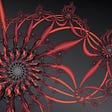## Probability Theory and Descriptive Statistics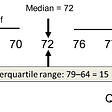## Tips and Tricks to Crack International Math Olympiad## Calculation## Accelerate quantum computation from a classical approach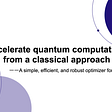## The world seen by the virus## Matthew Christopher Bartsh

I always follow back. I usually follow anyone who makes an interesting or okay response to one my articles. I often clap. I never give fewer than fifty claps.

## Part I: The Idea of Totality in Hegel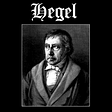## The Street of Crocodiles, Part 1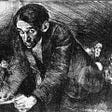## Life on… Venus?